# 1223989 static pushover analysis

29 Nov 2014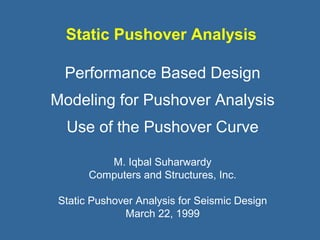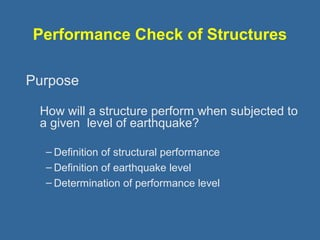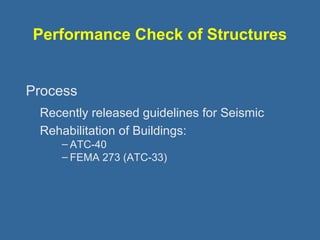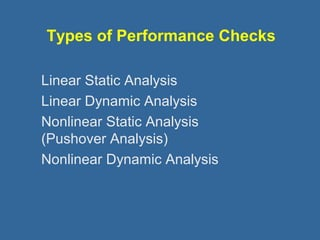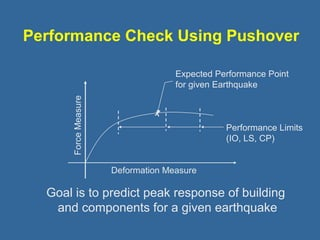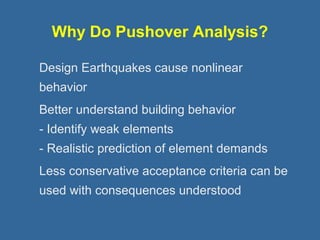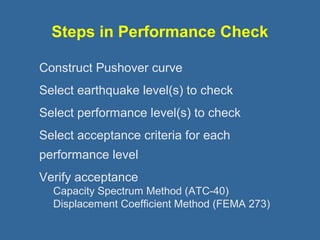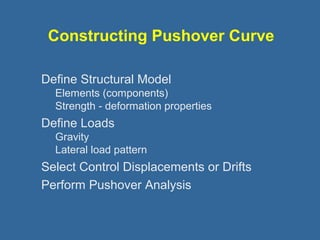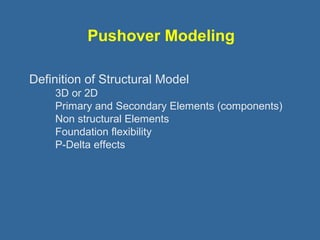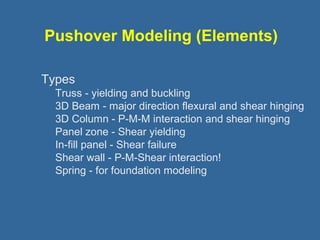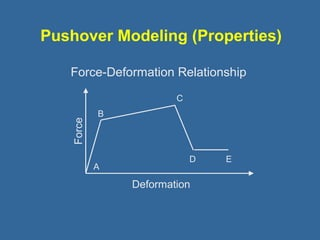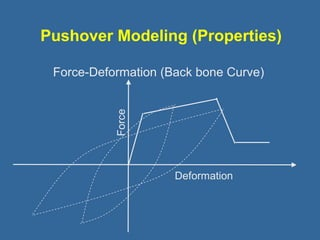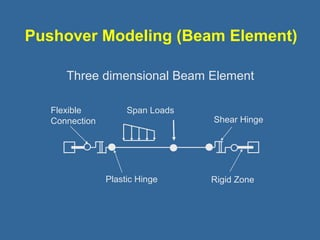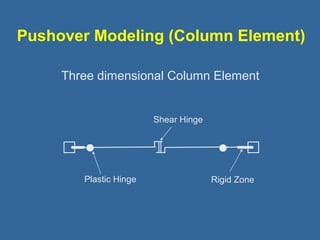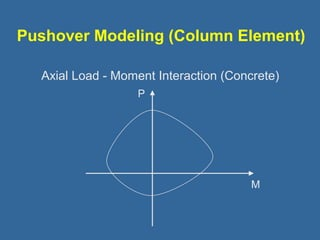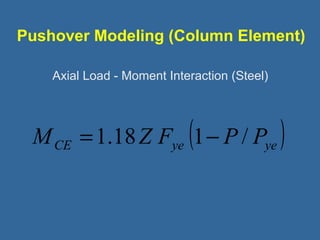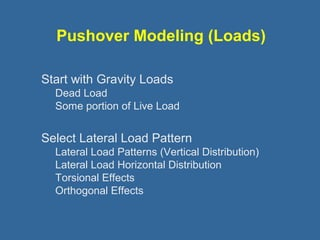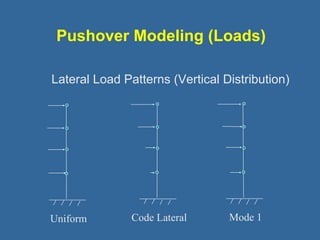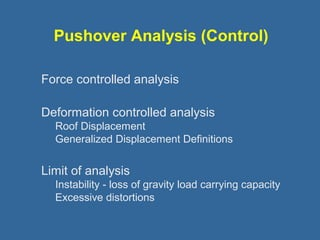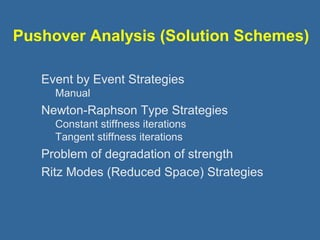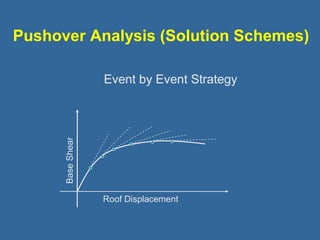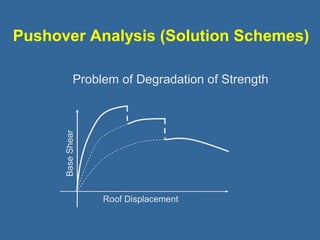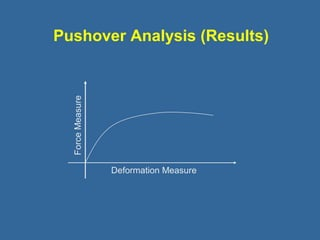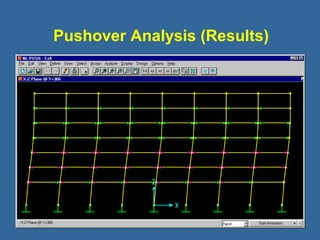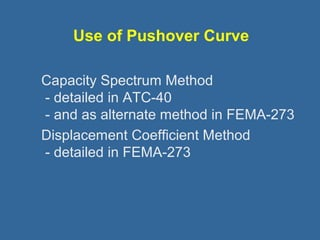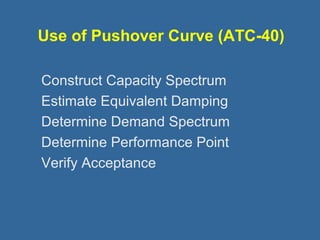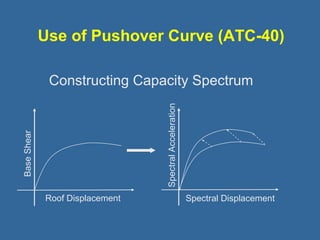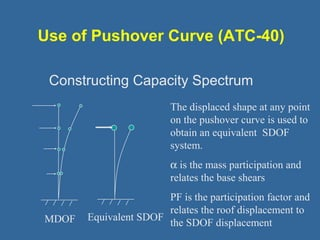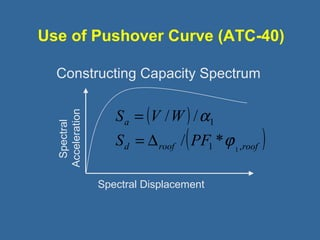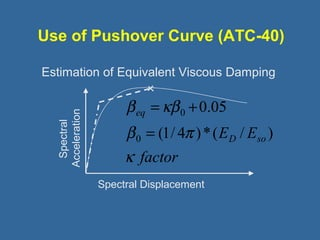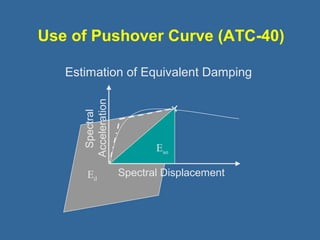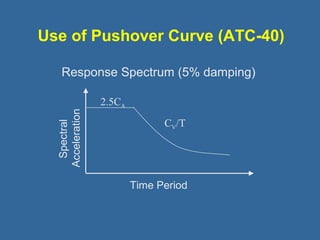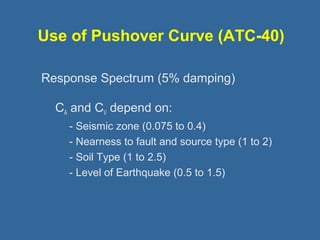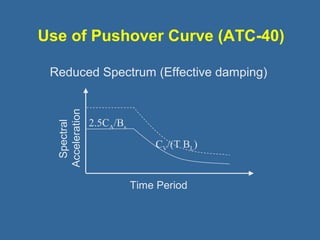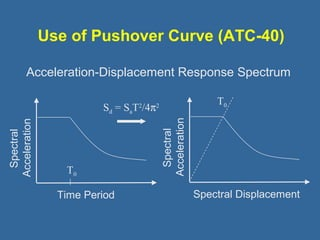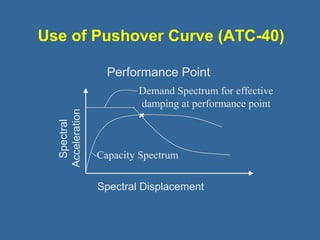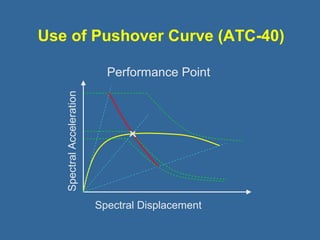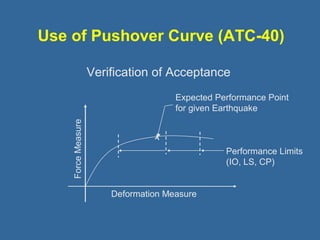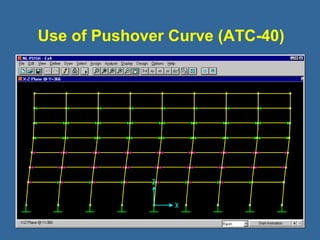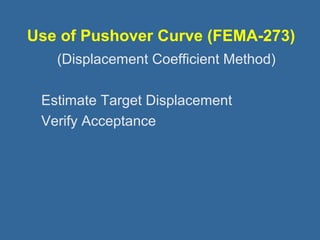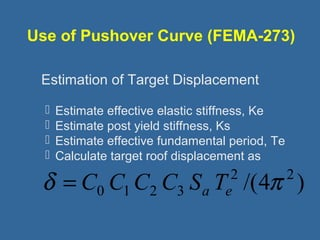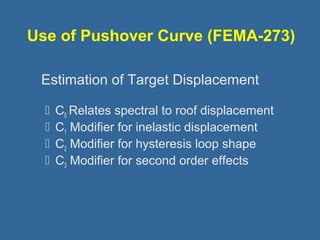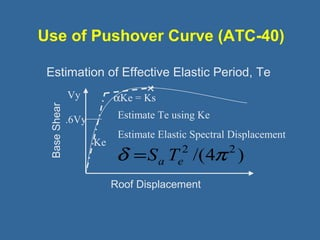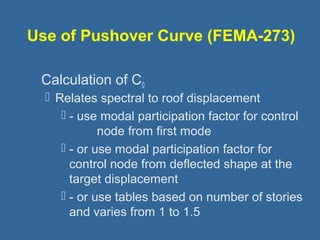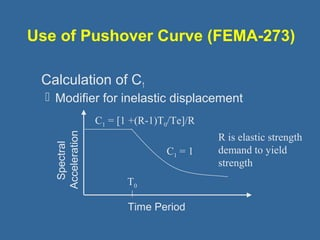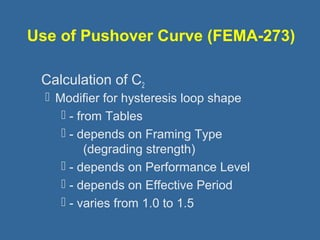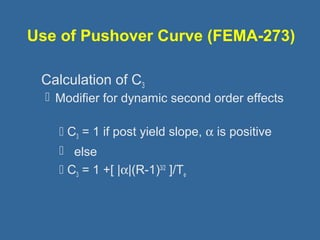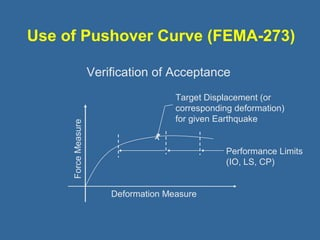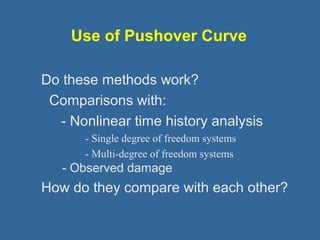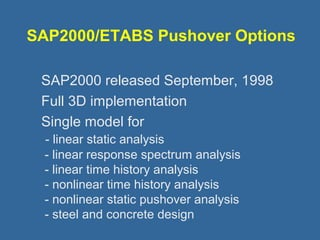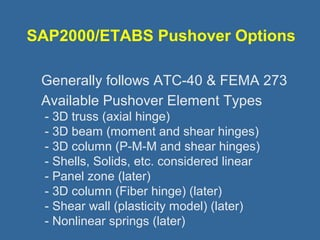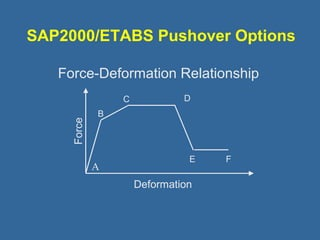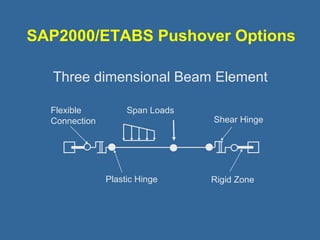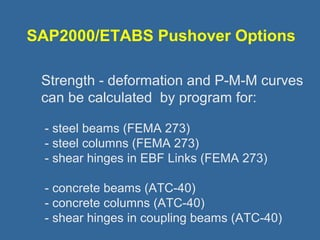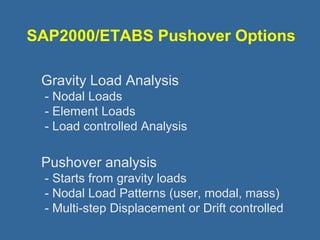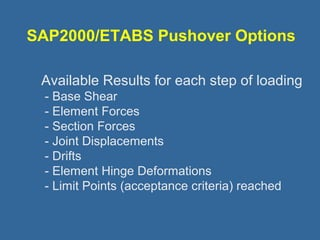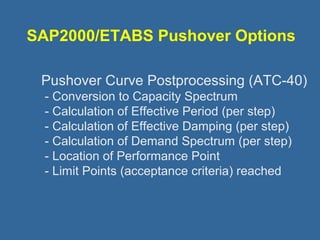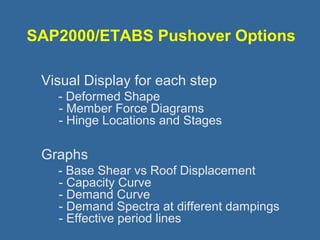1 sur 58

### 1223989 static pushover analysis

• 1. Static Pushover Analysis Performance Based Design Modeling for Pushover Analysis Use of the Pushover Curve M. Iqbal Suharwardy Computers and Structures, Inc. Static Pushover Analysis for Seismic Design March 22, 1999
• 2. Performance Check of Structures Purpose How will a structure perform when subjected to a given level of earthquake? – Definition of structural performance – Definition of earthquake level – Determination of performance level
• 3. Performance Check of Structures Process Recently released guidelines for Seismic Rehabilitation of Buildings: – ATC-40 – FEMA 273 (ATC-33)
• 4. Types of Performance Checks Linear Static Analysis Linear Dynamic Analysis Nonlinear Static Analysis (Pushover Analysis) Nonlinear Dynamic Analysis
• 5. Performance Check Using Pushover Expected Performance Point for given Earthquake Deformation Measure Force Measure Performance Limits (IO, LS, CP) Goal is to predict peak response of building and components for a given earthquake
• 6. Why Do Pushover Analysis? Design Earthquakes cause nonlinear behavior Better understand building behavior - Identify weak elements - Realistic prediction of element demands Less conservative acceptance criteria can be used with consequences understood
• 7. Steps in Performance Check Construct Pushover curve Select earthquake level(s) to check Select performance level(s) to check Select acceptance criteria for each performance level Verify acceptance Capacity Spectrum Method (ATC-40) Displacement Coefficient Method (FEMA 273)
• 8. Constructing Pushover Curve Define Structural Model Elements (components) Strength - deformation properties Define Loads Gravity Lateral load pattern Select Control Displacements or Drifts Perform Pushover Analysis
• 9. Pushover Modeling Definition of Structural Model 3D or 2D Primary and Secondary Elements (components) Non structural Elements Foundation flexibility P-Delta effects
• 10. Pushover Modeling (Elements) Types Truss - yielding and buckling 3D Beam - major direction flexural and shear hinging 3D Column - P-M-M interaction and shear hinging Panel zone - Shear yielding In-fill panel - Shear failure Shear wall - P-M-Shear interaction! Spring - for foundation modeling
• 11. Pushover Modeling (Properties) Force-Deformation Relationship B A C D E Force Deformation
• 12. Pushover Modeling (Properties) Force-Deformation (Back bone Curve) Force Deformation
• 13. Pushover Modeling (Beam Element) Three dimensional Beam Element Flexible Span Loads Connection Shear Hinge Plastic Hinge Rigid Zone
• 14. Pushover Modeling (Column Element) Three dimensional Column Element Shear Hinge Plastic Hinge Rigid Zone
• 15. Pushover Modeling (Column Element) Axial Load - Moment Interaction (Concrete) P M
• 16. Pushover Modeling (Column Element) Axial Load - Moment Interaction (Steel) CE ye ( ye ) M =1.18Z F 1- P / P
• 18. Pushover Modeling (Loads) Lateral Load Patterns (Vertical Distribution) Uniform Code Lateral Mode 1
• 19. Pushover Analysis (Control) Force controlled analysis Deformation controlled analysis Roof Displacement Generalized Displacement Definitions Limit of analysis Instability - loss of gravity load carrying capacity Excessive distortions
• 20. Pushover Analysis (Solution Schemes) Event by Event Strategies Manual Newton-Raphson Type Strategies Constant stiffness iterations Tangent stiffness iterations Problem of degradation of strength Ritz Modes (Reduced Space) Strategies
• 21. Pushover Analysis (Solution Schemes) Event by Event Strategy Roof Displacement Base Shear
• 22. Pushover Analysis (Solution Schemes) Problem of Degradation of Strength Roof Displacement Base Shear
• 23. Pushover Analysis (Results) Deformation Measure Force Measure
• 24. Pushover Analysis (Results)
• 25. Use of Pushover Curve Capacity Spectrum Method - detailed in ATC-40 - and as alternate method in FEMA-273 Displacement Coefficient Method - detailed in FEMA-273
• 26. Use of Pushover Curve (ATC-40) Construct Capacity Spectrum Estimate Equivalent Damping Determine Demand Spectrum Determine Performance Point Verify Acceptance
• 27. Use of Pushover Curve (ATC-40) Constructing Capacity Spectrum Roof Displacement Base Shear Spectral Displacement Spectral Acceleration
• 28. Use of Pushover Curve (ATC-40) Constructing Capacity Spectrum MDOF Equivalent SDOF The displaced shape at any point on the pushover curve is used to obtain an equivalent SDOF system. a is the mass participation and relates the base shears PF is the participation factor and relates the roof displacement to the SDOF displacement
• 29. Use of Pushover Curve (ATC-40) Constructing Capacity Spectrum Spectral Acceleration ( ) S = V W a S PF 1 d roof ( roof ) 1 / * Spectral Displacement 1 , / / j a = D
• 30. Use of Pushover Curve (ATC-40) Estimation of Equivalent Viscous Damping Spectral Acceleration b kb = + b p factor 0.05 Spectral Displacement ED Eso eq k (1/ 4 )*( / ) 0 0 =
• 31. Use of Pushover Curve (ATC-40) Estimation of Equivalent Damping Ed Eso Spectral Displacement Spectral Acceleration
• 32. Use of Pushover Curve (ATC-40) Response Spectrum (5% damping) Spectral Acceleration Time Period 2.5CA CV/T
• 33. Use of Pushover Curve (ATC-40) Response Spectrum (5% damping) CA and CV depend on: - Seismic zone (0.075 to 0.4) - Nearness to fault and source type (1 to 2) - Soil Type (1 to 2.5) - Level of Earthquake (0.5 to 1.5)
• 34. Use of Pushover Curve (ATC-40) Reduced Spectrum (Effective damping) Spectral Acceleration Time Period 2.5CA/Bs CV/(T BL)
• 35. Use of Pushover Curve (ATC-40) Acceleration-Displacement Response Spectrum Spectral Acceleration T0 S T0 d = SaT2/4p2 Time Period Spectral Acceleration Spectral Displacement
• 36. Use of Pushover Curve (ATC-40) Performance Point Spectral Acceleration Demand Spectrum for effective damping at performance point Capacity Spectrum Spectral Displacement
• 37. Use of Pushover Curve (ATC-40) Performance Point Spectral Acceleration Spectral Displacement
• 38. Use of Pushover Curve (ATC-40) Verification of Acceptance Expected Performance Point for given Earthquake Deformation Measure Force Measure Performance Limits (IO, LS, CP)
• 39. Use of Pushover Curve (ATC-40)
• 40. Use of Pushover Curve (FEMA-273) (Displacement Coefficient Method) Estimate Target Displacement Verify Acceptance
• 41. Use of Pushover Curve (FEMA-273) Estimation of Target Displacement  Estimate effective elastic stiffness, Ke  Estimate post yield stiffness, Ks  Estimate effective fundamental period, Te  Calculate target roof displacement as 2 /(4 2 ) d = C0 C1C2 C3 Sa Te p
• 42. Use of Pushover Curve (FEMA-273) Estimation of Target Displacement  C0 Relates spectral to roof displacement  C1 Modifier for inelastic displacement  C2 Modifier for hysteresis loop shape  C3 Modifier for second order effects
• 43. Use of Pushover Curve (ATC-40) Estimation of Effective Elastic Period, Te Vy .6Vy Base Shear Roof Displacement Ke aKe = Ks Estimate Te using Ke Estimate Elastic Spectral Displacement d =Sa Te2 /(4p 2 )
• 44. Use of Pushover Curve (FEMA-273) Calculation of C0  Relates spectral to roof displacement  - use modal participation factor for control node from first mode  - or use modal participation factor for control node from deflected shape at the target displacement  - or use tables based on number of stories and varies from 1 to 1.5
• 45. Use of Pushover Curve (FEMA-273) Calculation of C1  Modifier for inelastic displacement Spectral Acceleration C1 = [1 +(R-1)T0/Te]/R C1 = 1 T0 Time Period R is elastic strength demand to yield strength
• 46. Use of Pushover Curve (FEMA-273) Calculation of C2  Modifier for hysteresis loop shape  - from Tables  - depends on Framing Type (degrading strength)  - depends on Performance Level  - depends on Effective Period  - varies from 1.0 to 1.5
• 47. Use of Pushover Curve (FEMA-273) Calculation of C3  Modifier for dynamic second order effects  C3 = 1 if post yield slope, a is positive  else  C3 = 1 +[ |a|(R-1)3/2 ]/Te
• 48. Use of Pushover Curve (FEMA-273) Verification of Acceptance Target Displacement (or corresponding deformation) for given Earthquake Deformation Measure Force Measure Performance Limits (IO, LS, CP)